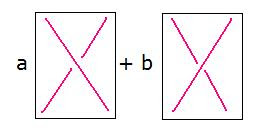occasional meanderings in physics' brave new world

Thursday, May 01, 2008

M Theory Lesson 179Recall that Bar-Natan's 1998 paper on the Grothendieck Teichmuller group discusses associated braids. On forgetting the crossing information, a braid in $B_{n}$ becomes a permutation on $n$ letters. So with bracketed endpoint sets, it marks a vertex of the $(n - 1)$ dimensional permutoassociahedron for $S_{n}$.

As well as the category of bracketed permutations, Bar-Natan considers the category of bracketed braids with linearised morphisms of the form $\sum \alpha_{j} B^{j}$, where the $B^{j}$ are allowable braids corresponding to a given element $P \in S_{n}$ and the $\alpha_{j}$ are numerical coefficients. For example, the Pauli permutation $\sigma_{x}$ gives morphisms of the formwhere $a$ and $b$ are usually rational numbers. The functor to the permutation category that forgets the braid structure is an example of a fibration of a very nice kind. The GT group is a certain group of endofunctors (from bracketed braids to bracketed braids) which fix $\sigma_{x}$ (with a choice of crossing). Bar-Natan shows that the bracketed braids are generated by the two basic diagrams shown above (ie. associator and $\sigma_{x}$ and inverses).CarlBrannen said...

Kea,

I'm guessing you're like me and using Microsoft Paint to draw diagrams. There are two secrets: (a) use the curve drawing tool, where you first draw the line, and then pick another point or two to modify it into a quadratic spline or whatever. They are very smooth.

(b) Don't save the image in jpg format, use png instead. It uses about the same amount of space but is much clearer.

May 02, 2008 5:03 PMKea said...

Carl, I know about the quadratic splines, and I use them a lot. But you might be right about using png. The fact is, I quite like the casual style.

May 02, 2008 5:13 PM